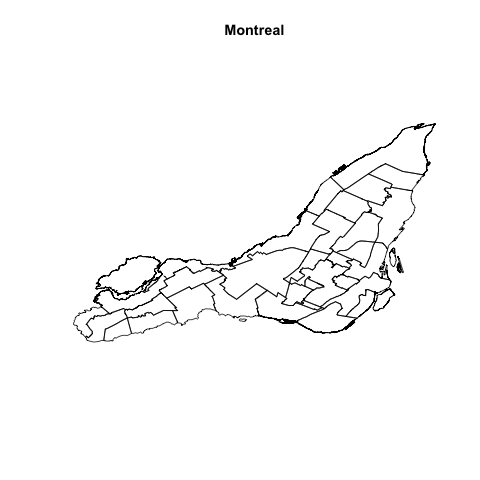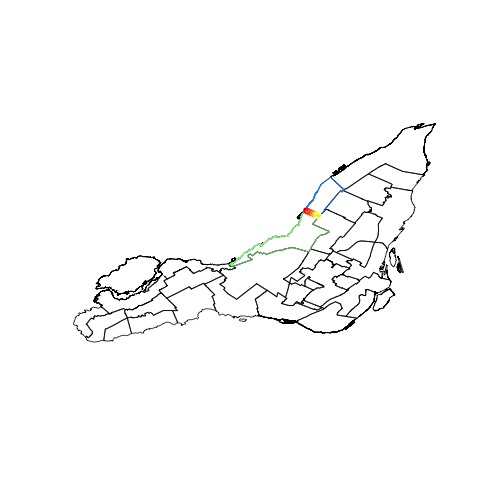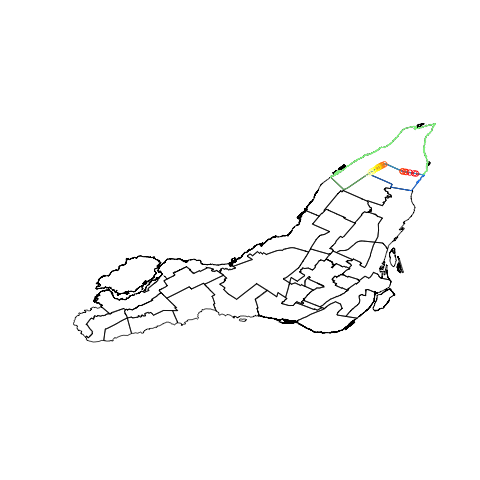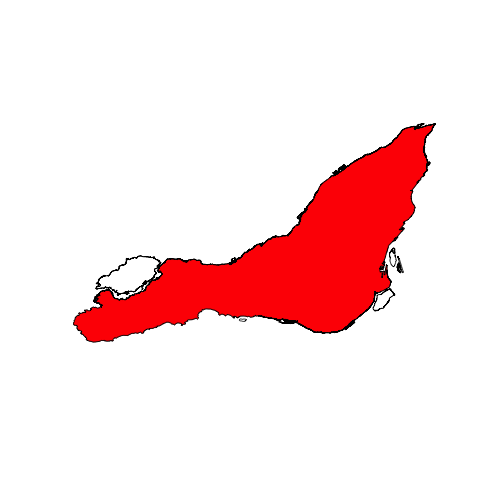After reading a really nice post about geo-marked bike accidents (Mapping Bike Accidents in R), I wanted to reuse the map for something else. Unfortunately, the shapefile used to create it does not give the entire border, but an aggregation of borough instead.

So, after a couple hour looking on the web for a simpler SHP file, I gave up and finally decided to create it myself. To do so, I used the SHP file given on the Mapping Bike Accidents post.mtl <- readShapePoly('montreal_borough_borders.shp')


The following lines are not elegant at all, but I didn’t know any better way to do the job.

So, basically, what is done here is quite simple:

1. find every borough that has any border delimiting Montreal ;
2. keep only the points I need

Since the object mtl is a spatial polygon data frame, I have to use this kind of syntax to get to the coordinates of the boroughs :


mtl@polygons[]@Polygons[]@coords


Here are some functions I need to find beginning and end points of the borough that are on the external frontier :


# plots the first borough in green, the second in blue, and each points from lIntersect
raccords=function(borough1,borough2,lIntersect){
plot(mtl)
lines(borough1,col="light green")
lines(borough2,col="dodger blue")
points(lIntersect,col=heat.colors(nrow(lIntersect)))
}
# Returns the position of lIntersect in borough
findPosition=function(borough,intersectPoint){
which(borough[,1]==intersectPoint & borough[,2]==intersectPoint)
}
# Help function to establish union between two bourough
raccords2=function(borough1,borough2){
plot(rbind(borough1,borough2),type="l")
lines(borough1,col="light green")
lines(borough2,lwd=2,col="dodger blue")
}


The idea is simple: the first point of a given borough should be the last of another one. This is what we try to find with the function raccords.


coords1=mtl@polygons[]@Polygons[]@coords
coords2=mtl@polygons[]@Polygons[]@coords
lIntersect=matrix(intersect(coords1,coords2),ncol=2)


The last instruction gives us 21 points. Let’s plot this.The point we want is the one on the West. Since colors are picked from a list created by the functionheat.colors(.), red ones arrive on top of the list. So the point we want it the first of the list. We only have to find it in both boroughs :


end1=findPosition(coords1,lIntersect[1,]) #end of coords1
beg2=findPosition(coords2,lIntersect[1,]) #beginning of coords2


For some districts, I encountered some issues.As you can see on the map, there is no intersection near the river (on the right). This is why I used the other raccord function, which helps finding the point in an heuristic way.

Once I found a beginning and an ending point for each district, I checked for possible error :


check=function(i){
plot(mtl)
coords=get(paste("coords",i,sep=""))
beg=get(paste("beg",i,sep=""))
end=get(paste("end",i,sep=""))
points(coords[beg:end,],col="red",cex=.1)
}
par(mfrow=c(4,4))
lapply(1:16,check)


The output stresses some issues. For some boroughs, what I expected to be an ending point was actually a beginning point. Hence, I had to use two different ways to finally create my polygon of Montreal.


assignCoord=function(i){
coords=get(paste("coords",i,sep=""))
beg=get(paste("beg",i,sep=""))
end=get(paste("end",i,sep=""))
assign(paste("co",i,sep="_"),coords[beg:end,],envir=globalenv())
}
lapply(c(1:2,4,7,10:15),assignCoord)
assignCoord2=function(i){
coords=get(paste("coords",i,sep=""))
beg=get(paste("beg",i,sep=""))
end=get(paste("end",i,sep=""))
assign(paste("co",i,sep="_"),coords[c(beg:nrow(coords),1:end),],envir=globalenv())
}
lapply(c(3,5,6,8,9,16),assignCoord2)
montreal=co_1
for(i in 2:17){
montreal=rbind(montreal,get(paste("co",i,sep="_")))
}


So here it is, my polygon of Montreal! If you want to check my R code, feel free.